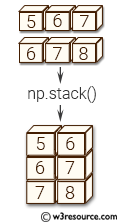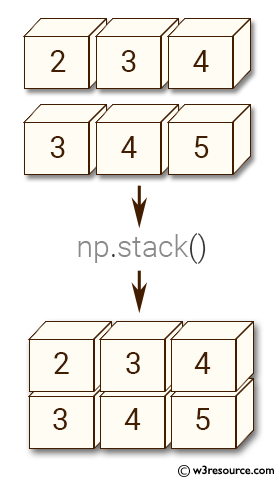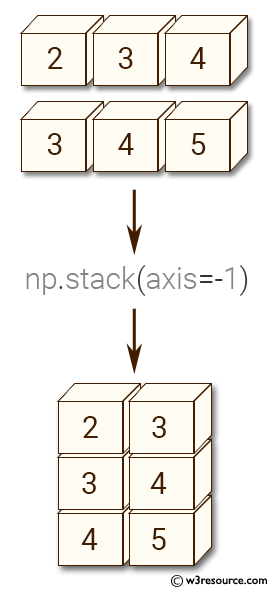# NumPy Array manipulation: stack() function

## numpy.stack() function

The stack() function is used to join a sequence of arrays along a new axis.
The axis parameter specifies the index of the new axis in the dimensions of the result. For example, if axis=0 it will be the first dimension and if axis=-1 it will be the last dimension.

Syntax:

`numpy.stack(arrays, axis=0, out=None)`Version: 1.15.0

Parameter:

Name Description Required /
Optional
arrays Each array must have the same shape. Required
axis The axis in the result array along which the input arrays are stacked. Optional
out If provided, the destination to place the result. The shape must be correct, matching that of what stack would have returned if no out argument were specified. Optional

Return value:

stacked : ndarray The stacked array has one more dimension than the input arrays.

Example-1: numpy.stack() function

``````>>> import numpy as np
>>> arrays = [np.random.randn(2, 3)for _ in range(8)]
>>> np.stack(arrays, axis=0).shape
(8, 2, 3)
``````

Example-2: numpy.stack() function

``````>>> import numpy as np
>>> np.stack(arrays, axis=1).shape
(2, 8, 3)
>>>
>>> np.stack(arrays, axis=2).shape
(2, 3, 8)
>>>
>>> x = np.array([2, 3, 4])
>>> y = np.array([3, 4, 5])
>>> np.stack((x, y))
array([[2, 3, 4],
[3, 4, 5]])
``````

Pictorial Presentation:Example-3: numpy.stack() function

``````>>> import numpy as np
>>> x = np.array([2, 3, 4])
>>> y = np.array([3, 4, 5])
>>> np.stack((x, y))
array([[2, 3, 4],
[3, 4, 5]])
>>> np.stack((x, y), axis=-1)
array([[2, 3],
[3, 4],
[4, 5]])
``````

Pictorial Presentation:Python - NumPy Code Editor:

Previous: concatenate()
Next: column_stack()

﻿